# Separation axiom

(diff) ← Older revision | Latest revision (diff) | Newer revision → (diff)
A condition imposed on a topological space, expressing the requirement that some disjoint (i.e. not having common points) sets can be topologically separated from each other in a specific way. The simplest (i.e. weakest) of these axioms apply only to one-point sets, i.e. to the points of a space. These are the so-called axioms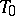(Kolmogorov's separation axiom, cf. also Kolmogorov space; Kolmogorov axiom) and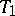. The next in line are(Hausdorff's separation axiom),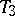(regularity axiom) and(normality axiom), which require, respectively, that every two different points (axiom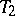), every point and every closed set not containing it (axiom), and every two disjoint closed sets (axiom) can be separated by neighbourhoods, i.e. are contained in disjoint open sets of the given space.
A topological space which satisfies the axiom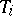,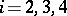, is called a-space; a-space is also called a Hausdorff space (cf. Hausdorff space), and a-space is called regular (cf. Regular space); a Hausdorff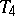-space is always regular and is called normal (cf. Normal space).
Functional separation is of particular significance. Two setsandin a given topological spaceare said to be functionally separated inif there exists a real-valued bounded continuous function, defined throughout the space, which takes one valueat all points of the set, and a value, different from, at all points of the set. It can always be supposed that,, and that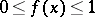at all points.
Two functionally-separable sets are always separable by neighbourhoods, but the converse is not always true. However, Urysohn's lemma holds: In a normal space, every two disjoint closed sets are functionally separable. A space in which every point is functionally separable from every closed set not containing it is called completely regular (cf. Completely regular space). A completely regular-space is called a Tikhonov space.